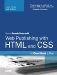User-Defined Functions

PHP enables you to create user-defined functions that, like JavaScript functions, enable you to package up code you want to reuse. Here's how a function is declared:

function myFunction(\$arg = 0) {   // Do stuff }

The function keyword indicates that you're creating a user-defined function. The name of the function follows. In this case, it's myFunction. The rules for function names and variable names are the samenumbers, letters, and underscores are valid. The list of arguments that the function accepts follows the function name, in parentheses.

The preceding function has one argument, \$arg. In this example, I've set a default value for the argument. The variable \$arg would be set to 0 if the function were called like this:

myFunction();

On the other hand, \$arg would be set to 55 if the function were called like this:

myFunction(55);

Functions can just as easily accept multiple arguments:

function myOtherFunction(\$arg1, \$arg2, \$arg3) {   // Do stuff }

As you can see, myOtherFunction accepts three arguments, one of which is an array. Valid calls to this function include the following:

myOtherFunction('one', 'two', array('three')); myOtherFunction('one', 'two'); myOtherFunction(0, 0, @stuff); myOtherFunction(1, 'blue');

One thing you can't do is leave out arguments in the middle of a list. So if you have a function that accepts three arguments, there's no way to set just the first and third arguments and leave out the second, or set the second and third and leave out the first. If you pass one argument in, it will be assigned to the function's first argument. If you pass in two arguments, they will be assigned to the first and second arguments to the function.

Returning Values

Optionally, your function can return a value, or more specifically, a variable. Here's a simple example of a function:

function add(\$a = 0, \$b = 0) {   return \$a + \$b; }

The return keyword is used to indicate that the value of a variable should be returned to the caller of a function. You could call the previous function like this:

\$sum = add(2, 3); // \$sum set to 5

A function can just as easily return an array. Here's an example:

function makeArray(\$a, \$b) {   return array(\$a, \$b); } \$new_array = makeArray('one', 'two');

If you don't explicitly return a value from your function, PHP will return the result of the last expression inside the function anyway. For example, let's say I wrote the add function like this:

function add(\$a = 0, \$b = 0) {  \$a + \$b; }

Because \$a + \$b is the last expression in the function, PHP will go ahead and return its result. That's the case for logical expressions as well. Here's an example:

function negate(\$a) {   !\$a; } negate(1); // returns false negate(0); // returns true

Your function can also return the result of another function, whether it's built in or one you wrote yourself. Here are a couple of examples:

function add(\$a = 0, \$b = 0) {   return \$a + \$b; } function alsoAdd(\$a = 0, \$b = 0) {   return add(\$a, \$b); }Sams Teach Yourself Web Publishing with HTML and CSS in One Hour a Day (5th Edition)
ISBN: 0672328860
EAN: 2147483647
Year: 2007
Pages: 305

Similar book on Amazon